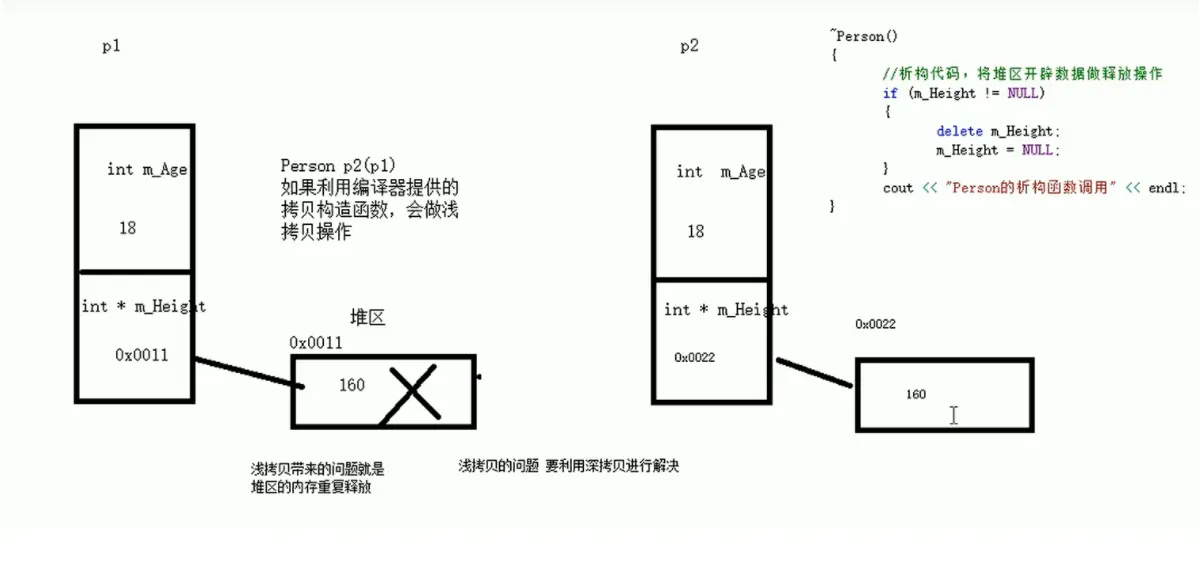# C++ 深拷贝与浅拷贝, 初始化列表，对象成员，静态成员相关分析

• 2022 年 8 月 05 日
• 本文字数：3172 字

阅读完需：约 10 分钟#### 深拷贝与浅拷贝

class Person {public:  //无参（默认）构造函数  Person() {    cout << "无参构造函数!" << endl;  }  //有参构造函数  Person(int age ,int height) {        cout << "有参构造函数!" << endl;    m_age = age;    m_height = new int(height);//使用new创建堆区数据，需要人为释放，new出来的东西是等到整个进程结束了才会自动释放。如果这个对象已经销毁，而这个类里没有析构函数却恰恰有个指针，自动释放的是栈区的变量，而不是堆区的，那么这个地址就没有指针指向它，就造成了内存泄漏。      }        //如果不利用深拷贝在堆区创建新内存，会导致浅拷贝带来的重复释放堆区问题： p1在进行有参初始化时，在堆区申请了一个空间，p1的height指针就指向这个空间，p2在进行拷贝初始化时使用的是编译器提供的浅拷贝，浅拷贝是对成员变量的简单赋值，指针传递的是地址，所以p2的height指针=p1的height指针,即两个height指针指向堆区的同一个地址，函数test01结束后，p1和p2把同一个空间释放了两次，所以程序崩了。    //既然编译器的浅拷贝不好用就自己写拷贝构造函数：  //拷贝构造函数    Person(const Person& p) {    cout << "拷贝构造函数!" << endl;    m_age = p.m_age;        //m_height = p.m_height;编译器的默认代码：浅拷贝。    m_height = new int(*p.m_height);      }  //析构函数  ~Person() {    cout << "析构函数!" << endl;    if (m_height != NULL)    {      delete m_height;    }  }public:  int m_age;  int* m_height;};void test01(){  Person p1(18, 180);  Person p2(p1);  cout << "p1的年龄： " << p1.m_age << " 身高： " << *p1.m_height << endl;  cout << "p2的年龄： " << p2.m_age << " 身高： " << *p2.m_height << endl;}int main() {  test01();  system("pause");  return 0;}

PS：#### 初始化列表

C++提供了初始化列表语法，用来初始化属性

class Person {public:  ////传统方式初始化  //Person(int a, int b, int c) {  //  m_A = a;  //  m_B = b;  //  m_C = c;  //}  //初始化列表方式初始化：  Person(int a, int b, int c) :m_A(a), m_B(b), m_C(c) {}//留出代码空间{}写其他的代码。  void PrintPerson() {    cout << "mA:" << m_A << endl;    cout << "mB:" << m_B << endl;    cout << "mC:" << m_C << endl;  }private:  int m_A;  int m_B;  int m_C;};int main() {  Person p(1, 2, 3);  p.PrintPerson();  system("pause");  return 0;}

#### 类对象作为类成员

C++类中的成员可以是另一个类的对象，我们称该成员为 对象成员

class A {}class B{    A a；}

B 类中有对象 A 作为成员，A 为对象成员

class Phone{public:  Phone(string name)  {    m_PhoneName = name;    cout << "Phone构造" << endl;  }  ~Phone()  {    cout << "Phone析构" << endl;  }  string m_PhoneName;};class Person{public:  //初始化列表可以告诉编译器调用哪一个构造函数  Person(string name, string pName) :m_Name(name), m_Phone(pName)  {    cout << "Person构造" << endl;  }  ~Person()  {    cout << "Person析构" << endl;  }  void playGame()  {    cout << m_Name << " 使用" << m_Phone.m_PhoneName << " 牌手机! " << endl;  }  string m_Name;  Phone m_Phone;};void test01(){  //当类中成员是其他类对象时，我们称该成员为 对象成员  //构造的顺序是 ：先调用对象成员的构造，再调用本类构造  //析构顺序与构造相反  Person p("张三" , "苹果X");  p.playGame();}int main() {  test01();  system("pause");  return 0;}

#### 静态成员

• 静态成员变量

• 所有对象共享同一份数据

• 在编译阶段分配内存

• 类内声明，类外初始化

• 静态成员函数

• 所有对象共享同一个函数

• 静态成员函数只能访问静态成员变量

**示例 1 ：**静态成员变量

class Person{  public:  static int m_A; //静态成员变量  //静态成员变量特点：  //1 在编译阶段分配内存，就是在exe运行前（代码区，全局区）就分配内存了，分配到全局区。  //2 类内声明，类外初始化（即必须有初始值，否则无法访问这块内存）  //3 所有对象共享同一份数据private:  static int m_B; //静态成员变量也是有访问权限的};int Person::m_A = 10;int Person::m_B = 10;//静态成员变量不属于某个对象上，所有对象共享一份数据，因此静态成员变量有两种访问方式。void test01(){  //静态成员变量两种访问方式  //1、通过对象（非静态成员变量只能通过类名的方法访问）  Person p1;  p1.m_A = 100;  cout << "p1.m_A = " << p1.m_A << endl;  Person p2;  p2.m_A = 200;  cout << "p1.m_A = " << p1.m_A << endl; //共享同一份数据  cout << "p2.m_A = " << p2.m_A << endl;  //2、通过类名（静态成员变量独有）  cout << "m_A = " << Person::m_A << endl;  //cout << "m_B = " << Person::m_B << endl; //私有权限访问不到}int main() {  test01();  system("pause");  return 0;}

**示例 2：**静态成员函数

class Person{public:  //静态成员函数特点：  //1 程序共享一个函数  //2 静态成员函数只能访问静态成员变量    static void func()  {    cout << "func调用" << endl;    m_A = 100;    //m_B = 100; //错误，不可以访问非静态成员变量。        //解释一：静态static成员函数它只属于类本身不属于每一个对象实例，独立存在。非静态成员，仅当实例化对象之后才存在。静态成员函数产生在前，非静态成员函数产生在后，静态函数无法访问一个不存在的东西。        //解释二：静态成员函数 不可以访问非静态成员变量，因为访问的时候不知道修改的是哪个对象的。由于m_A不属于某一个对象上的，所以可以访问。  }  static int m_A; //静态成员变量  int m_B; // private:  //静态成员函数也是有访问权限的  static void func2()  {    cout << "func2调用" << endl;  }};int Person::m_A = 10;void test01(){  //静态成员变量两种访问方式  //1、通过对象  Person p1;  p1.func();  //2、通过类名  Person::func();  //Person::func2(); //私有权限访问不到}int main() {  test01();  system("pause");  return 0;}### CtrlX

Pain is inevitable,suffering is optional 2022.08.01 加入

【个人网站】ctrlx.life 【联系方式】微信：gitctrlx 【软件技能】前端，C++，Python，研究网络工程，数据结构与算法。

## 评论